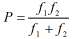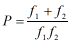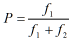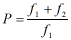Optics homework Help at TutorEye

## Optics:

Optics is a branch of physics, which deals with the different phenomena of light. It is associated with the propagation of light, its properties and its interaction with matters. Light exhibits dual behaviour as wave and particle. Physical optics deals with the wave nature of light and geometric optics deals with the particle nature of the light.

## OpticsSample Questions:

Question 1: A convex lens has 6 cm focal length and concave lens has -12 cm focal length. The focal length of the combination in contact will be

a) 12 cm            b) 6 cm

c) 10 cm            d) 24 cm

Explanation: Given,

Question 2: Two lenses of focal length f1 and f2 are in contact with each other. What is the equivalent power of a single lens formed by the combination of these two lenses?

a)b)c)d)Answer: b)Explanation: Power,

Question 3: A biconvex lens with equal radii curvature and refractive index 1.2 and focal length 20 cm. The radius curvature will be

a) 12 cm              b) 2 cm

c) 10 cm              d) 8 cm

Explanation: Given,

Question 4: If a convex lens of focal length f is cut along the line of the principal axis, what will be the new focal length of any half part?

a) 2f           b) 1.5f

c) f             d) 3f

Explanation: If the lens is cut along the principal axis

Question 5: Two lenses of power -10D and 5D are in contact with each other. The new focal length of the combination is

a) -10 cm             b) -20 cm

c) -15 cm             d) -25 cm

Explanation: Power of combination of lenses,

Question 6: Two thin lenses of focal lengths 50cm and -50cm are placed into contact with each other. What is the equivalent focal length of the combination?

a) ∞                    b) cannot be defined

c) zero               d) none

Explanation:  Given,

Question 7: A convex lens has a curved surface of radius of curvature R and refractive index n. If its plane surface is coated with silver, then it behaves as a

a) Convex lens             b) Concave lens

c) Concave mirror          d) Convex mirror

Explanation: It will

Question 8: A convex lens of focal length 20 cm is in contact with a concave lens of focal length 10 cm. What will be the power of combination?

a) -1.5 dioptres            b) 1.5 dioptres

c) 5 dioptres                 d) -5 dioptres

Explanation: Given,

Question 9: What is the resultant focal length of the combination, when thin convex and concave lenses of same focal lengths are put in contact with each other?

a) Zero                   b) One

c) Infinite              d) None of the above

Explanation: Focal length,

Question 10: The relationship between refractive index of the dielectric is given by

a) Snell’s law                  b) Newton’s law

c) Faraday’s law             d) None of these